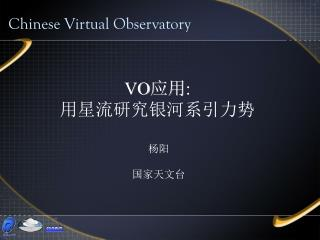# VO 应用 : 用星流研究银河系引力势 - PowerPoint PPT PresentationDownload PresentationVO 应用 : 用星流研究银河系引力势

VO 应用 : 用星流研究银河系引力势
Download Presentation## VO 应用 : 用星流研究银河系引力势

- - - - - - - - - - - - - - - - - - - - - - - - - - - E N D - - - - - - - - - - - - - - - - - - - - - - - - - - -
##### Presentation Transcript

1. Chinese Virtual Observatory VO应用: 用星流研究银河系引力势 杨阳 国家天文台

2. Outline • Scientific Background • Practice with VO tools VO-DAS TOPCAT • VO-DAS Application VO Tutorial @ CAS 2007

3. Scientific Background 恒星在银河系内的运动轨道由引力势决定 通过星流的运动轨迹，我们就可以估算出银河 系的引力势 方法： 1）在这个轨道上，我们选取BHB（蓝水平分支 星）恒星 BHB恒星距离更容易确定 VO Tutorial @ CAS 2007

4. VO Tutorial @ CAS 2007

5. 2）在双色图上确定多边形区域来选取BHB星的范围2）在双色图上确定多边形区域来选取BHB星的范围 BS BHB VO Tutorial @ CAS 2007

6. 确定BHB范围： 0.8<=u-g<=1.2； -0.3<=g-r<=0 u-g>=3*(g-r)+1.8 u-g>=g-r+1.3 u-g>=-0.33*(g-r)+1.1 VO Tutorial @ CAS 2007

7. 3）距离公式： g0-Mg+5 = 5 ㏒10d 其中 g0：视星等 Mg：绝对星等 （取0.7mag） d：距离 d=10^((g0-Mg+5)/5) VO Tutorial @ CAS 2007

8. 4）球面银道坐标到直角坐标系转换 银经（l），银纬（b），距离（d） X=d*cos(l*π/180 )cos(b* π /180)-8000 Y=d*sin(l* π /180 )cos(b* π /180) Z=d*sin（b* π /180) VO Tutorial @ CAS 2007

9. 5）可视化 VO Tutorial @ CAS 2007

10. Practice 1)在VO-DAS中选出A型星 VO-DAS同步方式查出满足条件的银经、银纬、视星等、u波段和r波段数据 ADQL语句： SELECT s.glon,s.glat,s.g,s.u,s.r FROM SDSS:Star2 s WHERE s.g>17.2 and s.g<20.2 and s.g- s.r>=-0.3 and s.g-s.r<=0 and s.u- s.g>=0.8 and s.u-s.g<=1.2 VO Tutorial @ CAS 2007

11. VO-DAS GUI客户端 VO Tutorial @ CAS 2007

12. VO-DAS查询数据结果 VO Tutorial @ CAS 2007

13. 2）结果数据传送给TOPCAT VO Tutorial @ CAS 2007

14. 3）TOPCAT中筛选出BHB星数据 u-4*g+3*r>=1.8 || u-2*g+r>=1.3 || u-0.67*g-0.33*r>=1.1 VO Tutorial @ CAS 2007

15. 图示BHB • 添加g-r，u-g字段 • 画密度图 VO Tutorial @ CAS 2007

16. 4）坐标转换可视化 VO Tutorial @ CAS 2007

17. 球面图 散点图 VO Tutorial @ CAS 2007

18. VO Tutorial @ CAS 2007

19. VO-DAS Application • 客户端 GUI、Command Line、Web Browser • 查询方式 异步查询、同步查询 VO Tutorial @ CAS 2007

20. Thank you! VO Tutorial @ CAS 2007